The radiant flux emitted from a point source in a certain direction per unit solid angle per unit projected area perpendicular to the specified direction is called radiance.
Radiance is denoted by Le,λ and it is equal to the double derivative of radiant flux with respect to projected surface area As and Solid angle ωs.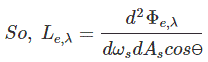where, ÆŸ is the angle between normal to the elemental and the given direction.
dAs is the elemental area and dωs is the elemental solid angle containing the given direction.
The unit of radiance is W/sr-m2.

In case of photometric quantity, the radiance is called as Luminance.
We can use the conversion equation to obtain luminance from radiance.Where, Km is the constant which is called maximum spectral luminous efficacy and its value is 683 lm/W.

So Luminance is the Luminous flux radiated from a point light source per unit solid angle and per unit projected area perpendicular to the specified direction.
Luminance is denoted byThe unit of Luminance is Lm/sr-m2 or Cd/m2.
If we take an analysis on the conservation of radiance and Luminance then we see that the radiance luminance or luminance from a source and the radiance and the luminance from the detector is the same i.e.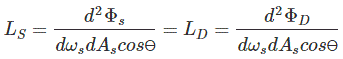It is because, if we consider that the radiation is neither gained or lost in the medium in which propagation of energy takes place between the source and the detector, then it must be that Φs = ΦD.
The luminance is a quantity that is conserved in the system.
Luminance is the same from the source and at the detector.
Luminance is neither source quantity nor detector quantity.
Luminance is purely geometric quantity of the beam connecting the source and the detector. The conservation of luminance is also true for presence of lenses or other optics.

The basic relationship between the luminance and the luminous flux is given below,
Φ = LG,
G is the geometric angle in steradian.

## Brightness is the Luminance

Luminance can’t be increased or decreased by any optical system. A system can only redirect the luminous flux. Suppose a page of a book has been considered with certain luminance. Then we can follow the equation below,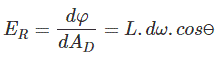Here, ER is the Illuminance on the book that redirects to our retina. This equation means that our eye converts the luminance into Illuminance on the retina. All the other detectors do the same as what the retina does. The retina reacts to the flux density of the radiant field, that is to the Illuminance. The principle physiological sensation of brightness is linked to the luminance of the source we are looking at.
The radiance and luminance has a relation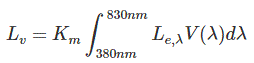Where, Km is the constant which is called maximum spectral luminous efficacy and its value is 683 lm/W.
Lv is luminance measured in cd/m2 and Le,λ is the radiance measured in W/m2-sr.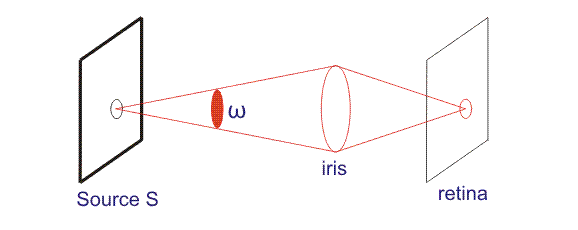Want To Learn Faster? 🎓
Get electrical articles delivered to your inbox every week.
No credit card required—it’s 100% free.